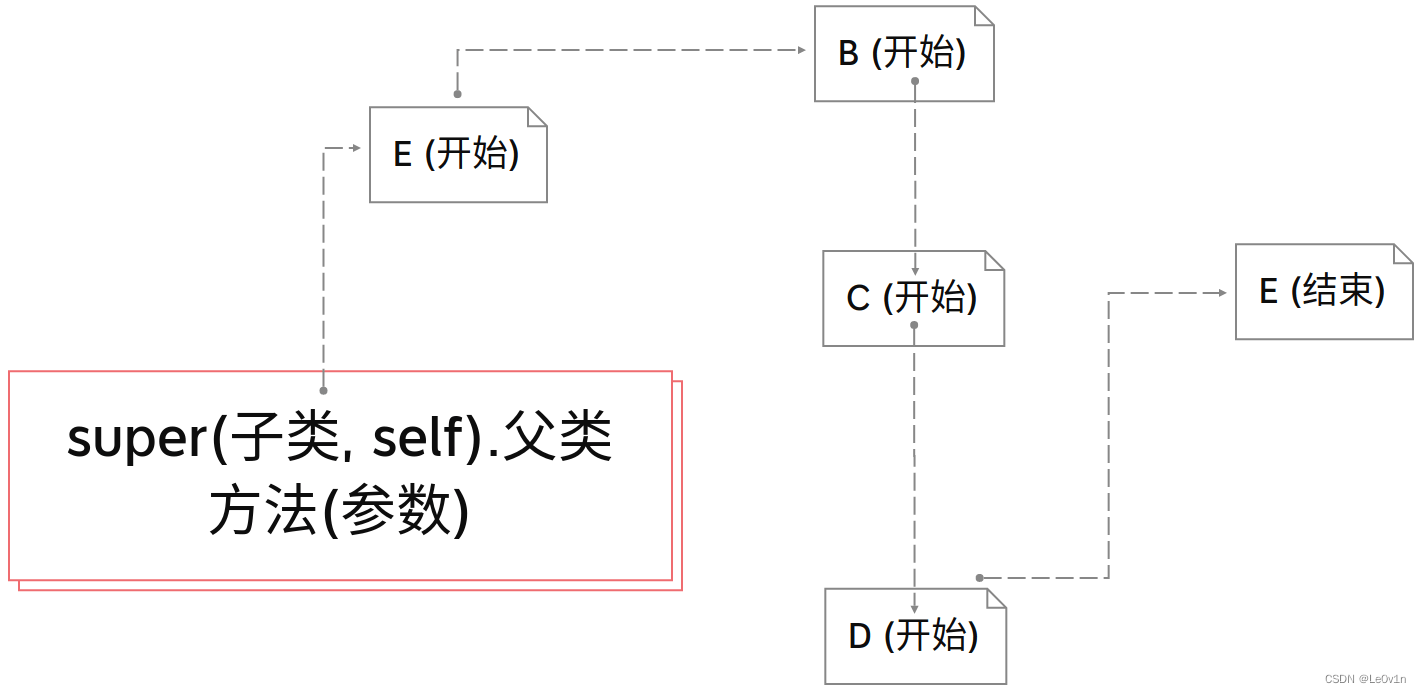# 0. 引言

Python中对象方法的定义很怪异，第一个参数一般都命名为`self`（相当于其它语言的`this`），用于传递对象本身，而在调用的时候则不必显式传递，系统会自动传递。

## 0.1 定义一个类

``````class A:
def method_1(self, msg):
print(msg)
return msg

a = A()
a.method_1(msg="Hello, class!")

"""
Hello, class!
"""
``````

## 0.2 在子类中调用父类的方法

``````class 子类(父类):
def 子类的方法(self, 参数):
父类.父类的方法(self, 参数)
``````

``````class A:
def method_parent(self, msg):
print(msg)
return msg

class B(A):
def method_child(self, msg):
# 父类.父类的方法(self, 参数)
A.method_parent(self, msg=msg)  # self必须写

b = B()
b.method_child(msg="Hello, class!")

"""
Hello, class!
"""
``````

# 1. super

``````class 子类(父类):
def 子类的方法(self, 参数):
super(子类，self).父类的方法(self, 参数)  # super中的self也必须写，但父类方法中不能写self
``````

``````class A:
def method_parent(self, msg):
print(msg)
return msg

class B(A):
def method_child(self, msg):
# super(自己这个类（子类）, self).父类的方法(参数)
super(B, self).method_parent(msg=msg)  # super中的self也必须写，但父类方法中不能写self

b = B()
b.method_child(msg="Hello, class!")

"""
Hello, class!
"""
``````

# 2. 两种方法的区别

## 2.1 `父类.父类方法(self, 参数)`

``````class A:
def __init__(self):
print("[开始] 访问A")
print("[结束] 访问A")

class B(A):
def __init__(self):
print("[开始] 访问B")
A.__init__(self)
print("[结束] 访问B")

class C(A):
def __init__(self):
print("[开始] 访问C")
A.__init__(self)
print("[结束] 访问C")

class D(A):
def __init__(self):
print("[开始] 访问D")
A.__init__(self)
print("[结束] 访问D")

class E(B, C, D):  # 多继承
def __init__(self):
print("[开始] 访问E")
B.__init__(self)
C.__init__(self)
D.__init__(self)
print("[结束] 访问E")

# 实例化类E
e = E()

"""
[开始] 访问E
[开始] 访问B
[开始] 访问A
[结束] 访问A
[结束] 访问B
[开始] 访问C
[开始] 访问A
[结束] 访问A
[结束] 访问C
[开始] 访问D
[开始] 访问A
[结束] 访问A
[结束] 访问D
[结束] 访问E
"""
``````

## 2.2 `super(子类, self).父类方法(参数)`

``````class A:
def __init__(self):
print("[开始] 访问A")
print("[结束] 访问A")

class B(A):
def __init__(self):
print("[开始] 访问B")
super(B, self).__init__()
print("[结束] 访问B")

class C(A):
def __init__(self):
print("[开始] 访问C")
super(C, self).__init__()
print("[结束] 访问C")

class D(A):
def __init__(self):
print("[开始] 访问D")
super(D, self).__init__()
print("[结束] 访问D")

class E(B, C, D):  # 多继承
def __init__(self):
print("[开始] 访问E")
super(E, self).__init__()
print("[结束] 访问E")

# 实例化类E
e = E()

"""
[开始] 访问E
[开始] 访问B
[开始] 访问C
[开始] 访问D
[开始] 访问A
[结束] 访问A
[结束] 访问D
[结束] 访问C
[结束] 访问B
[结束] 访问E
"""
``````

`super机制`里可以保证公共父类仅被执行一次，至于执行的顺序，是按照MRO（Method Resolution Order）：方法解析顺序进行的。

## 2.3 图解两种方法

### 2.3.1 `父类.父类方法(self, 参数)`### 2.3.2 `super(子类, self).父类方法(参数)`# 3. 例子讲解`super(子类, self).__init__()`

## 3.1 作用

`super().__init__()`，就是继承父类的`__init__()`方法，同样可以使用`super()`去继承其他方法。

## 3.2 三种继承的对比 —— 彻底搞懂 `super(子类, self).__init__()`方法

``````# 定义父类
# 定义父类
class Parent:
# 定义父类的初始化方法
def __init__(self, param_parent="父类的参数"):
self.param_parent = param_parent  # 将初始化需传入的参数定义为类的attr

# 定义父类的方法
def function_parent(self):
print("这是一个父类方法！")

class Child(Parent):
# 这里重新定义了__init__()方法就说明该方法将覆盖从父类继承的__init__方法
def __init__(self, param_parent):  # 即便你不使用父类的参数，但在初始化的时候也要写上！
# 调用父类的__init__()方法而已
# super(Child, self).__init__()  # 这里__init__()函数里面的值要写，不然就被父类的默认值覆盖了！
super(Child, self).__init__(param_parent=param_parent)  # 这里__init__()函数里面的值要写，不然就被父类的默认值覆盖了！
"""
1. super(Child, self).__init__() -> 结果：父类的参数
2. super(Child, self).__init__(param_parent=param_paren) -> 结果：因为又继承了父类的__init__方法，所以需传入该参数（如果父类有默认值，也可以不传）
"""
def function_child(self):
super(Child, self).function_parent()  # 这句话的意思就是，在调用子类function_child时，该方法会继承父类的funtion_parent，也就是调用子类 -> 去父类找方法 -> 父类的方法执行 -> 回到子类看看还没有别的代码要执行

# 实例化子类
aaa = Child(param_parent="因为又继承了父类的__init__方法，所以需传入该参数（如果父类有默认值，也可以不传）")
print(aaa.param_parent)
aaa.function_child()  # Child -> Parent -> Parent -> Child

"""
因为又继承了父类的__init__方法，所以需传入该参数（如果父类有默认值，也可以不传）
这是一个父类方法！
"""
``````

``````# 定义父类
class A:
# 定义父类的初始化方法
def __init__(self, param_parent="父类默认的参数"):
self.param_parent = param_parent

# 定义父类的方法
def function_parent(self, msg_parent):
print(f"[父类A的方法] message: {msg_parent}")

# 1. [完全继承] 定义子类（继承父类A）
class B(A):
pass  # 完全继承（包括属性和方法）

# 2. [重写__init__方法，剩下的都继承] 定义子类（继承A）
class C(A):
def __init__(self, param_child):
self.param_child = param_child  # 重新了__init__方法

# 3. [在__init__方法中添加了子类独有的参数，且仍然继承父类的属性和方法]
class D(A):
def __init__(self, param_parent, param_child):
self.param_child = param_child
# 重新继承父类的__init__方法
super(D, self).__init__(param_parent=param_parent)

# 实例化子类B, C, D
b = B()  # 没有传入值，所以使用父类A中的默认值
c = C(param_child="C的参数")
d = D(param_parent="父类A的参数（是需要自己定义的,也可以使用默认参数（如果有缺省的话））", param_child="子类D特有的参数")

"""
调用子类的attr和方法
"""
# 1. [完全继承] 定义子类（继承父类A）
# attr
print(f"[父类A的属性] B.param_parent: {b.param_parent}")
# function
b.function_parent(msg_parent="子类B直接使用父类A的方法")  # B -> A -> A -> B
print("\n")

# 2. [重写__init__方法，剩下的都继承] 定义子类（继承A）
# attr
try:
print(f"[父类A的属性] C.param_parent: {c.param_parent}")  # 因为C重写了__init__方法，因此父类的attr被覆盖了，所以不能调用！
except:
print("[报错！] 因为C重写了__init__方法，因此父类的attr被覆盖了，所以不能调用！")
print(f"[子类C特有的属性] C.param_child: {c.param_child}")  # 重写后的attr，当然可以调用
# function
c.function_parent(msg_parent="子类C直接使用父类A的方法")  # C -> A -> A -> C
print("\n")

# 3. [在__init__方法中添加了子类独有的参数，且仍然继承父类的属性和方法]
# attr
print(f"[父类A的属性] D.param_parent: {d.param_parent}")  # 虽然D重写了__init__方法，但最该方法末尾又继承了父类的attr，所以可以直接调用
print(f"[子类D特有的属性] D.param_child: {d.param_child}")  # 重写后的attr，当然可以调用
# function
d.function_parent(msg_parent="子类D直接使用父类A的方法")  # D -> A -> A -> D

"""
[父类A的属性] B.param_parent: 父类默认的参数
[父类A的方法] message: 子类B直接使用父类A的方法

[报错！] 因为C重写了__init__方法，因此父类的attr被覆盖了，所以不能调用！
[子类C特有的属性] C.param_child: C的参数
[父类A的方法] message: 子类C直接使用父类A的方法

[父类A的属性] D.param_parent: 父类A的参数（是需要自己定义的,也可以使用默认参数（如果有缺省的话））
[子类D特有的属性] D.param_child: 子类D特有的参数
[父类A的方法] message: 子类D直接使用父类A的方法
"""
``````

## 3.3 小例子

``````class A:
def __init__(self, param_parent):
# 将传入的参数param_parent存储到实例中
self.param_parent = param_parent

def function_parent(self, msg_parent):
# 直接使用传进的参数而不存到实例中
print(f"[父类]message: {msg_parent}")
return msg_parent

class B(A):  # B继承自A
def __init__(self, param_parent, param_child):  # 不管用没用到父类__init__方法中的参数，都得写上
# 调用父类的初始化方法
super(B, self).__init__(param_parent=param_parent)
# 将传入的参数param_child存储到实例中
self.param_child = param_child

# 定义类方法
def function_child(self, msg_child):
print(f"[子类]message: {msg_child}")
# 再调用父类的方法
super(B, self).function_parent(msg_parent=msg_child)
return msg_child

# 实例化子类B并传入__init__所需参数
b = B(param_child="子类的参数", param_parent="父类的参数")

# 调用这个类的attr
print(f"self.param_child: {b.param_child}")

# 因为继承了父类的__init__()方法，所以子类也有父类的实例变量(但参数是我们自己传入的)
print(f"self.param_parent: {b.param_parent}")

# 调用这个类的方法
b.function_child(msg_child="[子类的方法] 传入的信息")

# 调用父类的方法
b.function_parent(msg_parent="[父类的方法] 传入的信息")

"""
self.param_child: 子类的参数
self.param_parent: 父类的参数
[子类]message: [子类的方法] 传入的信息
[父类]message: [子类的方法] 传入的信息
[父类]message: [父类的方法] 传入的信息
"""
``````

# 4. 定义类时的建议

1. 子类在重写`__init__`方法时，要把父类`__init__`方法中的参数都写上（有默认值的可以不写），不然会报错
2. 因为可能涉及到继承，建议在写`__init__()`方法时，将里面的参数都写上默认值，这样继承的时候就不用那么麻烦了
3. 类的继承只是继承了类的模板（attr和方法），但一般不继承它里面的值（默认值除外）

# 参考

1. https://www.jb51.net/article/198478.htm
2. https://blog.csdn.net/a__int__/article/details/104600972